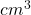## Find the density of each of these materials. A brick measures 23 cm by 10 cm and is 5 cm deep. It has a mass of 2242.5 g.<

Question

Find the density of each of these materials.
A brick measures 23 cm by 10 cm
and is 5 cm deep.
It has a mass of 2242.5 g.
density of brick =
A slab of marble measures 50 cm by 80 cm
and is 2.1 cm thick.
It has a mass of 22176 g.
density of marble =
g/cm3
g/cm3

in progress 0
2 months 2021-07-25T16:48:28+00:00 1 Answers 2 views 0

V= 23x10x5

V= 1150D=m/v

D=2242/1150

D of the brick = 1.949 g/V= 50x80x2.1

V= 8400

D=22176/8400

D of the marble = 2.64 g/